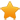Switch Editions?
CancelChannel: CodeSection,代码区,网络安全 - CodeSec
Viewing all articles

# ggedit 0.0.2: a GUI for advanced editing of ggplot2 objects

0
0

Last week the updated version of ggedit was presented in RStudio::conf2017 . First, a BIG thank you to the whole RStudio team for a great conference and being so awesome to answer the insane amount of questions I had (sorry!). For a quick intro to the package see theprevious post.

To install the package:

devtools::install_github(&quot;metrumresearchgroup/ggedit&quot;,subdir=&quot;ggedit&quot;)

Highlights of the updated version. verbose script handling during updating in the gagdet (see video below) verbose script output for updated layers and theme to parse and evaluate in console or editor colourpicker control for both single colours/fills and and palletes output for scale objects eg scale*grandient,scale*grandientn and scale*manual verbose script output for scales eg scale*grandient,scale*grandientn and scale*manual to parse and evaluate in console or editor input plot objects can have the data in the layer object and in the base object. ggplot(data=iris,aes(x=Sepal.Width,y=Sepal.Length,colour=Species))+geom_point() ggplot(data=iris,aes(x=Sepal.Width,y=Sepal.Length))+geom_point(aes(colour=Species)) ggplot()+geom_point(data=iris,aes(x=Sepal.Width,y=Sepal.Length,colour=Species)) plot.theme(): S3 method for class ‘theme’ visualizing theme objects in single output visual comparison of two themes objects in single output will be expanded upon in upcoming post RStudio::conf2017 Presentation #devtools::install_github("metrumresearchgroup/ggedit",subdir="ggedit")
rm(list=ls())
library(ggedit)
#?ggedit
p0=list(
Scatter=iris%>%ggplot(aes(x =Sepal.Length,y=Sepal.Width))+
geom_point(aes(colour=Species),size=6),
ScatterFacet=iris%>%ggplot(aes(x =Sepal.Length,y=Sepal.Width))+
geom_point(aes(colour=Species),size=6)+
geom_line(linetype=2)+
facet_wrap(~Species,scales='free')+
labs(title='Some Title')
)
#a=ggedit(p.in = p0,verbose = T) #run ggedit
dat_url <- paste0("https://raw.githubusercontent.com/metrumresearchgroup/ggedit/master/RstudioExampleObj.rda")
ldply(a,names) ## .id V1 V2
## 1 UpdatedPlots Scatter ScatterFacet
## 2 UpdatedLayers Scatter ScatterFacet
## 3 UpdatedLayersElements Scatter ScatterFacet
## 4 UpdatedLayerCalls Scatter ScatterFacet
## 5 updatedScales Scatter ScatterFacet
## 6 UpdatedScalesCalls Scatter ScatterFacet
## 7 UpdatedThemes Scatter ScatterFacet
## 8 UpdatedThemeCalls Scatter ScatterFacet plot(a)

comparePlots=c(p0,a\$UpdatedPlots)
names(comparePlots)[c(3:4)]=paste0(names(comparePlots)[c(3:4)],"Updated") Initial Comparison Plot plot(as.ggedit(comparePlots))

Apply updated theme of first plot to second plot comparePlots\$ScatterFacetNewTheme=p0\$ScatterFacet+a\$UpdatedThemes\$Scatter
plot(as.ggedit(comparePlots[c("ScatterFacet","ScatterFacetNewTheme")]),
plot.layout = list(list(rows=1,cols=1),list(rows=2,cols=1))
)

#Using Remove and Replace Function ##Overlay two layers of same geom (comparePlots\$ScatterMistake=p0\$Scatter+a\$UpdatedLayers\$ScatterFacet[])

Remove (comparePlots\$ScatterNoLayer=p0\$Scatter%>%
rgg(oldGeom = 'point'))

Replace Geom_Point layer on Scatter Plot (comparePlots\$ScatterNewLayer=p0\$Scatter%>%
rgg(oldGeom = 'point',
oldGeomIdx = 1,
newLayer = a\$UpdatedLayers\$ScatterFacet[]))

Remove and Replace Geom_Point layer and add the new theme (comparePlots\$ScatterNewLayerTheme=p0\$Scatter%>%
rgg(oldGeom = 'point',
newLayer = a\$UpdatedLayers\$ScatterFacet[])+
a\$UpdatedThemes\$Scatter)

Cloning Layers A geom_point layer (l=p0\$Scatter\$layers[]) ## mapping: colour = Species
## geom_point: na.rm = FALSE
## stat_identity: na.rm = FALSE
## position_identity Clone the layer (l1=cloneLayer(l)) ## mapping: colour = Species
## geom_point: na.rm = FALSE
## stat_identity: na.rm = FALSE
## position_identity all.equal(l,l1) ##  TRUE Verbose copy of layer (l1.txt=cloneLayer(l,verbose = T)) ##  "geom_point(mapping=aes(colour=Species),na.rm=FALSE,size=6,data=NULL,position=\"identity\",stat=\"identity\",show.legend=NA,inherit.aes=TRUE)" Parse the text (l2=eval(parse(text=l1.txt))) ## mapping: colour = Species
## geom_point: na.rm = FALSE
## stat_identity: na.rm = FALSE
## position_identity all.equal(l,l2) ##  TRUE Back to our example #Original geom_point layer
parse(text=cloneLayer(p0\$ScatterFacet\$layers[],verbose = T)) ## expression(geom_point(mapping = aes(colour = Species), na.rm = FALSE,
## size = 6, data = NULL, position = "identity", stat = "identity",
## show.legend = NA, inherit.aes = TRUE)) #new Layer
parse(text=a\$UpdatedLayerCalls\$ScatterFacet[]) ## expression(geom_point(mapping = aes(colour = Species), na.rm = FALSE,
## size = 3, shape = 22, fill = "#BD2020", alpha = 1, stroke = 0.5,
## data = NULL, position = "identity", stat = "identity", show.legend = NA,
## inherit.aes = TRUE))

Jonathan Sidi joined Metrum Researcg Group in 2016 after working for several years on problems in applied statistics, financial stress testing and economic forecasting in both industrial and academic settings.

To learn more about additional open-source software packages developed by Metrum Research Group please visit the Metrum website .

Viewing all articles

More Pages to Explore .....